# Trigonometric Functions

The trigonometric ratios can also be considered as functions of a variable which is the measure of an angle.

This angle measure can either be given in degrees or radians . Here, we will use radians. Since any angle with a measure greater than $2\pi$ radians or less than $0$ is equivalent to some angle with measure $0\le \theta <2\pi$ , all the trigonometric functions are periodic .

The graph of the sine function looks like this: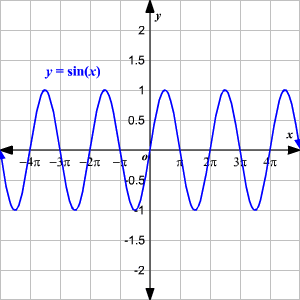Note that the domain of the function $y=\mathrm{sin}\left(x\right)$ ) is all real numbers (sine is defined for any angle measure), the range is $-1\le y\le 1$ .

The graph of the cosine function looks like this: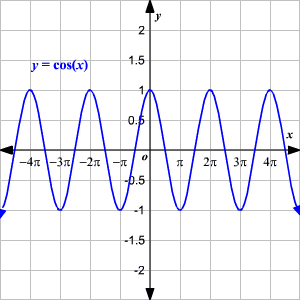The domain of the function $y=\mathrm{cos}\left(x\right)$ is all real numbers (cosine is defined for any angle measure), the range is $-1\le y\le 1$ .

The graph of the tangent function looks like this: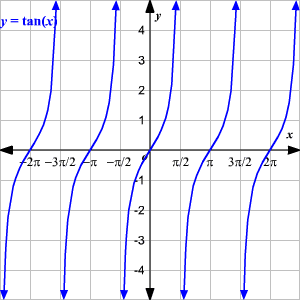The domain of the function $y=\mathrm{tan}\left(x\right)$ ) is all real numbers except the values where $\mathrm{cos}\left(x\right)$ is equal to $0$ , that is, the values $\frac{\pi }{2}+\pi n$ for all integers $n$ . The range of the tangent function is all real numbers.

The graph of the secant function looks like this: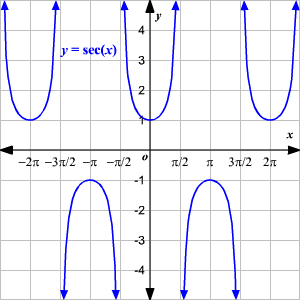The domain of the function $y=\mathrm{sec}\left(x\right)=\frac{1}{\mathrm{cos}\left(x\right)}$ is again all real numbers except the values where $\mathrm{cos}\left(x\right)$ is equal to $0$ , that is, the values $\frac{\pi }{2}+\pi n$ for all integers $n$ . The range of the function is $y\le -1$ or $y\ge 1$ .

The graph of the cosecant function looks like this: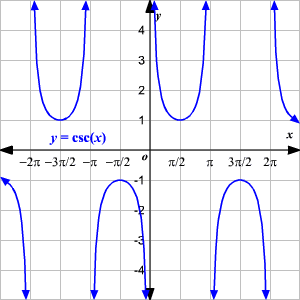The domain of the function $y=\mathrm{csc}\left(x\right)=\frac{1}{\mathrm{sin}\left(x\right)}$ is all real numbers except the values where $\mathrm{sin}\left(x\right)$ is equal to $0$ , that is, the values $\pi n$ for all integers $n$ . The range of the function is $y\le -1$ or $y\ge 1$ .

The graph of the cotangent function looks like this: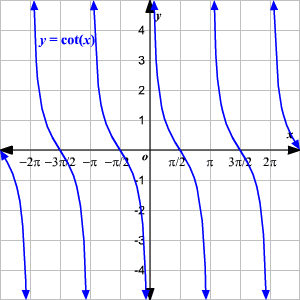The domain of the function $y=\mathrm{cot}\left(x\right)=\frac{\mathrm{cos}\left(x\right)}{\mathrm{sin}\left(x\right)}$ is all real numbers except the values where $\mathrm{sin}\left(x\right)$ is equal to $0$ , that is, the values $\pi n$ for all integers $n$ . The range of the function is all real numbers.